# i want the derivation of volume of 'terahedron'

11 years ago

dear sachin

The tetrahedron has 4 faces, 4 vertices, and 6 edges. Each face is an equilateral triangle. Three faces meet at each vertex.

Begin with a tetrahedron of edge length s. Its faces are equilateral triangles. The length of their sides is s, and the measure of their interior angles is p/3.

First, find the area of each triangular face. Multiply that by the number of faces to get the total surface area, A.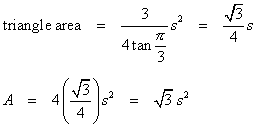The dihedral angle formula can be applied here because three faces meet at each vertex. All of the faces are equilateral triangles, so let a b = g = p/3.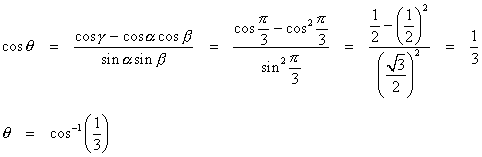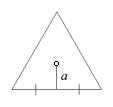Find the apothem of a face, and use it in the calculations for the inradius and circumradius.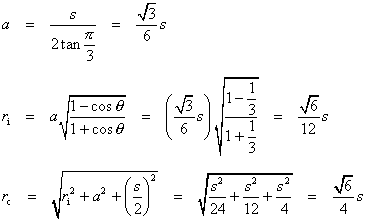Now, the volume formula.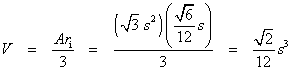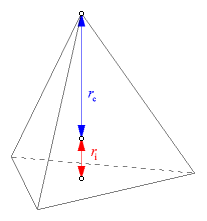Here is another way to work out the volume. The tetrahedron is also a pyramid, and its height is the sum of the inradius and the circumradius. Use that fact and apply the pyramid volume formula. Redundant calculations like this are a good way of checking the results.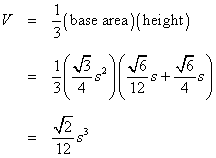Please feel free to post as many doubts on our discussion forum as you can.we will get you the answer and detailed  solution very  quickly.

All the best.

Regards,
Prudhvi Teja

Now you can win exciting gifts by answering the questions on Discussion Forum. So help discuss any query on askiitians forum and become an Elite Expert League askiitian